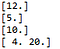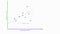Machine Learning seems to be very complex when you look at all the math, statistics and various algorithms involved with it, but with the rise and development of many open source packages like TensorFlow, the daunting task of building machine learning models seem much easier than before. TensorFlow was originally developed by researchers and engineers from the Google Brain team within Google’s AI organization, it comes with strong support for machine learning and deep learning and the flexible numerical computation core is used across many other scientific domains.

So, in today’s blog post we will learn about the basics of TensorFlow and try to implement a simple linear regression model without breaking our head too much about all the mathematical mambo-jumbo involved in it. Although you won’t understand the true meaning of what “breaking your head” means unless you try to work out the math behind a deep learning model for pattern recognition and interpreting the results produced by it. Wink Wink!

But don’t lose courage. Stay true to your path and as a great man once said: “Nothing ventured, Nothing gained!” ,although that’s just a dialogue from the main character of my favourite anime Steins;Gate but it is quite motivational indeed! Right?

Well let’s not go off topic now and move ahead.

TensorFlow uses the concept of Tensors which can be treated as basic units of data. The structure of a Tensor is similar and can be compared to that of an array of some primitive values. A tensor can be defined as an N-Dimensional vector which can be represented through a multidimensional array.

The concept of nodes and graphs

While creating a model with TensorFlow there are 2 major steps involved, the first one is to create a graph like structure and organize the nodes in it. After that is done we simply need to run the graph.

So, there are basically 3 different types of nodes which are used to make these graphs : Constant, Variable, and Placeholder nodes.

A Constant Node contains constant values which cannot be changed.

A Variable Node can be used to store some initial values, but we can change them if we want to, later on. A special function is required to initialize the values in the beginning.

Placeholder Nodes don’t need to have any particular values defined initially but can be assigned values when running sessions. Below we have a code snippet which shows how these nodes actually work.The output for the above code

In line 6 we see how a constant node is defined. We can also define a node in the way we did in line 7 without explicitly mentioning the data type of the node. It is very easy to understand the output of the addition of the constant nodes. We can also see how we can define the variable nodes and change their values as and when we want. The initial value of the variable node is given as [5.0] in line 17 but we change its value to [10.0] in line 24.

We will be using the placeholder nodes a lot and it is crucial that we understand them in a better manner. Unlike the constant or variable nodes we don’t provide any particular data which a placeholder has to hold when defining them. We define another operator node to multiply the values of these placeholder nodes once their values are provided. In line 35 we provide the value of the placeholder nodes in the form of 2 arrays and the output that we get is the element-wise multiplication of the 2 arrays.

Linear Regression

So, in the next part of our tutorial we will now see how we can implement a Linear Regression model using TensorFlow.

Imagine we have a function y=mx+c. Here y is the dependent variable and x is the independent variable. Hence, the change in variable x produces a change in variable y. So, in a linear regression task our job is to find the appropriate values of the slope m and the intercept value c so that we can get an accurate estimated value of y for any given x.Getting a best fit line for the data points

To get the proper values for the slope and intercept we initially assume some dummy values for the slope and intercept and find out an estimated solution for y with respect to x. We find the squared error between the estimated value of y and the actual value of y. In machine learning terminology the slope and the intercept are widely known as the weights and bias. We will be using this terminology from now on for referring them. Our aim is to minimize the squared error term as much as we can by finding the appropriate values of the slope and intercept. This is achieved through a process called Gradient Descent.

Now we aren’t going too much into the mathematics involved as I already promised you previously, but a proper understanding of the topic is required and I would appreciate you, doing some research yourself.

So, let’s start coding then, we import the TensorFlow package as follows:

We then define values in the form of x_train and y_train. The values of y_train will alter with respect to the values of x_train. The aim of our model is to provide a resultant array which will have values very close to that of y_train. We have also defined 2 placeholder nodes which we will be using later. We also define the values of our weight and bias terms. It is always advised to keep their values small initially.

Next, we define our linear model as lm= Wx+b which works the same as the previously defined y=mx+c. Using the values defined for x_train and y_train, it would mean that if a graph was plotted it would be similar to something like the one given below, where clearly the value of W should be -1 and the value of b should be 1. Hence, our aim is to create a model which can come close to achieving those values.

We calculate the overall loss and use Gradient Descent with a learning rate of 0.01 and try to minimize the loss function. It is advised that the learning rate shouldn’t be too large otherwise it will take a lot of time to converge or too small because then reaching the final result takes a lot of time.

We now define our session and run it. We train our model for 1000 iterations for minimizing the loss.

So, we finally see that after training for 1000 iterations we have a model which uses values of weight and bias very close to -1 and 1 and the output provided by the model is very close to [-4,-5,-6,-7]. This means that we have successfully built our linear regression model!

You can congratulate yourself now! You’ve done a great job and learnt something new. Now, the next thing you can do is choose more arbitrary values for y and x and try to train a model yourself.

You can take a look at the whole code below:

Written by

Data Driven Investor

from confusion to clarity, not insanity

Welcome to a place where words matter. On Medium, smart voices and original ideas take center stage - with no ads in sight. Watch
Follow all the topics you care about, and we’ll deliver the best stories for you to your homepage and inbox. Explore
Get unlimited access to the best stories on Medium — and support writers while you’re at it. Just \$5/month. Upgrade